# Daily Challenge #87

Hello Koders, This daily challenge is easy. In this daily challenge, you will return the place value of the number like:-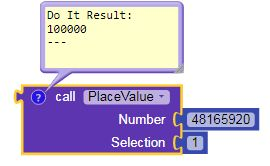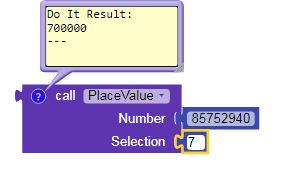Another example:-
Number: 56466672
Selection: 46
Result-46000

The input could be decimal number.

If there are multiple selection number, it will choose the first one like: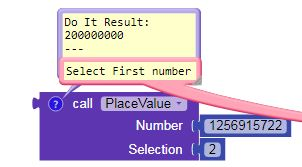## Errors

All input should be numbers. it could also be text or anything.If there are any errors like: selected number is 0, selected number doesn’t exist, number & selection inputs are not number etc etc it will return 0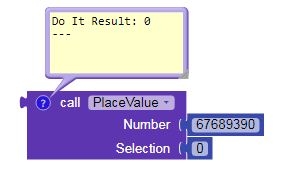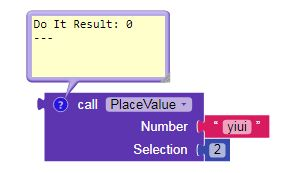## Rules

Only blocks, no extensions are allowed. It will have to work in both, companion and apk

## Solution

I will tick the solution to the best answer. The best answer should be easy in lowest blocks possible.

Place Value
What is Place Value?

In math, every digit in a number has a place value.

Place value can be defined as the value represented by a digit in a number on the basis of its position in the number.

In 13548, 1 is in ten thousands place and its place value is 10,000,
3 is in thousands place and its place value is 3,000,
5 is in hundreds place and its place value is 500,
4 is in tens place and its place value is 40,
8 is in ones place and its place value is 8.

Understanding the place value of digits in numbers helps in writing numbers in their expanded form. For instance, the expanded form of the number above, 13548 is 10,000 + 3,000 + 500 + 40 + 8.

A place value chart can help us in finding and comparing the place value of the digits in numbers through millions. The place value of a digit increases by ten times as we move left on the place value chart and decreases by ten times as we move right.

In 3287263, 3 is in millions place and its place value is 3000000,
2 is hundred thousands place and its place value is 200000,
8 is in ten thousands place and its place value is 80000,
7 is in thousands place and its place value is 7000,
2 is in hundreds place and its place value is 200,
6 is in ten place and its place value is 60,
3 is in ones place and its place value is 3.

The place value of digits in numbers can also be represented using base-ten blocks and can help us write numbers in their expanded form.

## Good Luck!! Try to answer this Daily Challenge

If you have any problem understanding, tell me

4 Likes

Great DC! But i need to have dinner now.
Come back later!

3 Likes

Can the input “number” be decimal number? If not I will have to debug this.
And can the selection be 2 or more than?

Yes… It can be

I have two question. You mean all can be true?

Yes… True

One more thing…
If number=7843184.12; selection=43
Will the output be 43000.00
or 43000
or 40000?

Number=7843184.12; selection=43
Result=43000.00
Number=7843184.1; selection=43
Result=43000.0
Number=7843184; selection=43
Result=43000

Clear???

1 Like

ok sure I am clear now
I was confused before cuz you didn’t mention it in your first post.

1 Like

Anyone here, nothing why?
Ontstudios, Yoshi, WatermelonIce, vknow360 where are they?

I try so hard, but there still some errors in my blocks, and I just leave from school just now.